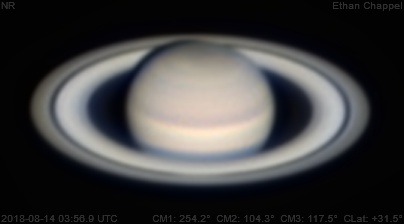# Saturn 2018-08-14 03:56 UTC

CM1: 254.20°

CM2: 104.30°

CM3: 117.50°

CLat: +31.50°

Description

Seeing remained average as I moved on to imaging Saturn. I had a harder time processing this image since I only had one video of the planet to process.

Notes:

• The northern storm may be rising.

Equipment

Celestron C8 EdgeHD

ZWO ASI224MC

Tele Vue 2.5x Powermate

Logs
```FireCapture v2.6  Settings
------------------------------------
Observer=Ethan Chappel
Location=Cibolo, TX, USA
Scope=Celstron C14 EdgeHD
Camera=ZWO ASI224MC
Filter=RGB
Profile=Saturn
Diameter=17.67"
Magnitude=0.30
CMI=254.2° CMIII=117.4°  (during mid of capture)
FocalLength=3050mm (F/8)
Resolution=0.25"
Filename=2018-08-14-0356_9-EC-RGB-Sat.ser
Date=2018_08_14
Start=035543.274
Mid=035658.279
End=035813.285
Start(UT)=035543.274
Mid(UT)=035658.279
End(UT)=035813.285
Duration=150.011s
Date_format=yyyy_MM_dd
Time_format=HHmmss
LT=UT -6h
Frames captured=4998
File type=SER
Binning=no
Bit depth=8bit
Debayer=yes
DebayerAlgorithm=NearestNeighbor
BayerPattern=RG
ROI=272x196
ROI(Offset)=0x0
FPS (avg.)=33
Shutter=30.00ms
Gain=350 (58%)
HardwareBin=off
HighSpeed=off
AutoGain=off
WRed=52 (off)
AutoHisto=75 (off)
WBlue=95 (off)
FPS=100 (off)
Gamma=50 (off)
USBTraffic=100 (off)
AutoExposure=off
Brightness=1 (off)
SoftwareGain=10 (off)
Histogramm(min)=0
Histogramm(max)=58
Histogramm=22%
Noise(avg.deviation)=0.41
Limit=150 Seconds
Sensor temperature=33.5°C
```